Next: Notch filter Up: Z-plane, causality, and feedback Previous: Laurent expansion

# INTRODUCTION TO ALL-PASS FILTERS

An all-pass filter'' is a filter whose spectral magnitude is unity.

Given an input X(Z) and an output Y(Z), we know that the spectra of the two are the same, i.e.,.The existence of an infinitude of all-pass filters tells us that an infinitude of wavelets can have the same spectrum. Wave propagation without absorption is modeled by all-pass filters. All-pass filters yield a waveform distortion that can be corrected by methods discussed in chapter.

The simplest example of an all-pass filter is the delay operator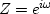itself. Its phase as a function ofis simply.

A less trivial example of phase distortion can be constructed from a single root Zr, where Zr is an arbitrary complex number. The ratio of any complex number to its complex conjugate, say (x+iy)/(x-iy), is of unit magnitude, because, taking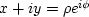and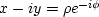,the ratio is.Thus, given a minimum-phase filter,we can take its conjugate and make an all-pass filter P(Z) from the ratio.A simple case is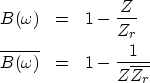(45) (46)
The all-pass filteris not causal because of the presence of 1/Z in.We can repair that by multiplying by another all-pass operator, namely, Z. The resulting causal all-pass filter is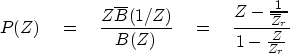(47)
Equation (47) can be raised to higher powers to achieve a stronger frequency-dispersion effect. Examples of time-domain responses of various all-pass filters are shown in Figure 13.

 disper Figure 13 Examples of causal all-pass filters with real poles and zeros. These have high frequencies at the beginning and low frequencies at the end.The denominator of equation (47) tells us that we have a pole at Zr. Let this location be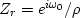.The numerator vanishes at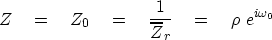(48)
In conclusion, the pole is outside the unit circle, and the zero is inside. They face one another across the circle at the phase angle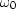.

The all-pass filter (47) outputs a complex-valued signal, however. To see real outputs, we must handle the negative frequencies in the same way as the positive ones. The filter (47) should be multiplied by another like itself but withreplaced by; i.e., with Zr replaced by.The result of this procedure is shown in Figure 14.allpass
Figure 14
All-pass filter with a complex pole-zero pair. The pole and zero are at equal logarithmic distances from the unit circle.

A general form for an all-pass filter is,where A(Z) is an arbitrary minimum-phase filter. That this form is valid can be verified by checking that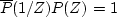.

## EXERCISES:

1. Verify thatfor the general form of an all-pass filter P(Z) =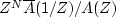.
2. Given an all-pass filter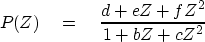with poles at Zp=2 and Zp=3, what are b, c, d, e, and f?Next: Notch filter Up: Z-plane, causality, and feedback Previous: Laurent expansion
Stanford Exploration Project
10/21/1998